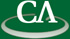The Computer Algebra Group (CAG) at Simon Fraser University began in 1997. Members of the group are primarilly intested in the area known as Computer Algebra or Symbolic Computation. We use and develop software for the Maple and the Magma computer algebra systems. The CAG maintains a close relationship with Waterloo Maple, Inc., the producer of the computer algebra system Maple. The director of the CAG, Dr. Michael Monagan, is one of authors of Maple. The current areas of research interest in our group include Simplification of algebraic formulae. Polynomial factorization and polynomial GCD computation. Symbolic summation and integration. Groebner bases and ideal theoretic computations. Symbolic solution of algebraic, ordinary and partial differential equations. High precision numerical differentation and integration. Numerical solution of analytic functions. Numerical solution of ordinary and partial differential equations. Recovery of formulae from floating point approxmations. Visualization of graphs, eigenvectors, vector fields, etc. Linear algebra over the integers and finite fields. Several members of CAG are participating in the Mathematics of Computer Algebra and Analysis Project of The Mathematics of Information Technology and Complex Systems (MITACS), a federally funded Network of Centres of Excellence (NCE). Back to the CAG Home Page.